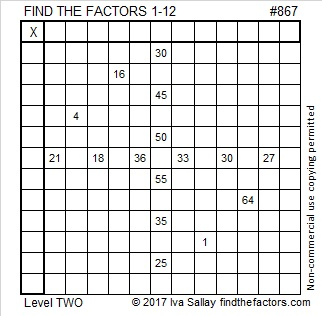# 867 and Level 2

867 is composed of three consecutive numbers so 867 is divisible by 3. The middle number of those three numbers, 6, 7, 8 is 7 so 867 is NOT divisible by 9.Print the puzzles or type the solution on this excel file: 12 factors 864-874

867 is the hypotenuse of two Pythagorean triples:

• 483-720-867, which is 3 times (161-240-289)
• 408-765-867 which is (8-15-17) times 51

867 is 300 in BASE 17 because 3(17²) = 867.

• 867 is a composite number.
• Prime factorization: 867 = 3 × 17 × 17, which can be written 867 = 3 × 17²
• The exponents in the prime factorization are 1 and 2. Adding one to each and multiplying we get (1 + 1)(2 + 1) = 2 × 3  = 6. Therefore 867 has exactly 6 factors.
• Factors of 867: 1, 3, 17, 51, 289, 867
• Factor pairs: 867 = 1 × 867, 3 × 289, or 17 × 51
• Taking the factor pair with the largest square number factor, we get √867 = (√289)(√3) = 17√3 ≈ 29.44486This site uses Akismet to reduce spam. Learn how your comment data is processed.